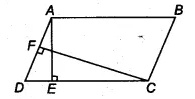Q

# In Fig. 9.15, ABCD is a parallelogram, AE ⊥ DC and CF ⊥ AD. If AB = 16 cm, AE = 8 cm and CF = 10 cm, find AD.

Q : 1       In Fig. , ABCD is a parallelogram, and . If  and , find AD.ViewsWe have,
AB = 16 cm, AE = 8 cm and CF = 10 cm

Since ABCD is a parallelogram,
therefore, AB = DC = 16 cm
We know that, area of parallelogram (ABCD) = base . height
= CD  AE  = (16  8 )